Courses

# Scott Connected Transformers Electrical Engineering (EE) Notes | EduRev

## Electrical Engineering (EE) : Scott Connected Transformers Electrical Engineering (EE) Notes | EduRev

The document Scott Connected Transformers Electrical Engineering (EE) Notes | EduRev is a part of the Electrical Engineering (EE) Course Electrical Machines.
All you need of Electrical Engineering (EE) at this link: Electrical Engineering (EE)

Scott Connected Transformers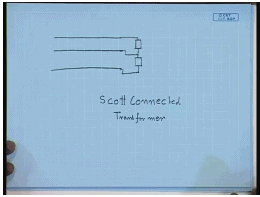In many situations it becomes necessary to supply a balanced or unbalanced two phase or three single phase load on a balanced three phase supply. One way to do, let us say for a two phase load to connect the two phases between two lines, this is one possibility; however, even when these phases are balanced impedances are balanced, they will draw unbalanced current from the supply. Not only that many two phase loads will require a balanced two phase supply as well.

Some of this two phase loads are large art furnaces or induction melting furnaces. The power can range in megawatts. So, care should be taken to make sure that the loading of the three phase system is balanced to the extent possible. This can be done by what is called a Scott connected transformer. This Scott connected transformer can convert a balanced three phase supply to a balanced two phase supply. If the load on the two phase supply is balanced, then the line current drawn from the three phase will also be balanced, we will see how?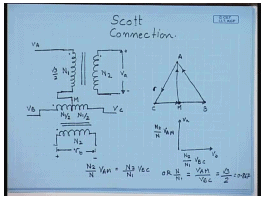For Scott connection, we take two single phase transformers; let us say here the turns is N. This turn is N 2, this is the dot polarity; another transformer with a 50 percent tapping, so that this number of turns is N 1 by 2 and this is N 1 by 2, and the corresponding secondary have the same number of turns N 2. Now let us connect this end of one of the transformers to the 50 percent tapping point and keep balanced three phase supply to this side; that is V A, V B and V C. And let us see what happens to the induced voltages here. This is the instantaneous polarity here; here this is the instantaneous polarity.

So, the three phase supply is balanced. So, let this point be M. So, let us say this is the phasor V b C and this is the phasor A, so that this is the phasor V a B; this is the phasor V c A. Now the voltage phasor V m C, V m C will be half of V b C. This is the point M V m C, and what will be the voltage phasor V a M? V a M will be V a C minus V m C, V a C minus V M C; V a C is in this direction, this is V M C. So, the voltage phasor V a M will be this. It shows that the voltage phasor V a M is at right angle to the voltage phasor V b C. Hence, the induced voltage V a will be in phase with the voltage V a M.

So, this will be the voltage V a, and its magnitude will be N 2 by N into V a M, whereas the voltage phasor V b will be in phase with the voltage V b C. So, this will be the voltage phasor V b, and its magnitude will be N 2 by N 1 into V b C. Now if we want the voltages V a B and V b C to be balanced two phase that is they are already 90 degree, and if we want their magnitudes also to be same, then it is imperative that we should have N 2 by N into V a M should be equal to N 2 by N 1 into V b C. Or we want N by N 1 should be equal to V a M by V b C. Now from this phasor diagram V a M by V b C equal to root 3 by 2 or equal to 0.867. Hence, number of turns should be here root 3 by 2 into N 1.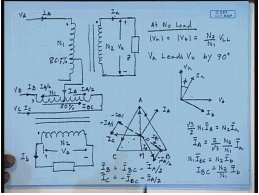So, a Scott connected transformer can be obtained by two single phase transformers with turns N 1 and N 2. The first term any suitable turns ratio N 1 by N 2 with one transformer having a tapping at 86.7 percent, the other transformer having a tapping at 50 percent. Now we connect the 86.7 percent tapping to the 50 percent tapping, and then give three phase balanced supply in this manner V A, V B, V C in which case we have just seen that the induced voltages V a and V B. This instantaneous polarity we have seen at no load mod of V a equal to mod of V b equal to N 2 by N 1 into V L L of the primary, and V a leads V b by 90 degree. So, this is at no load.

Now let us see what happens. So, at no load we have got a balanced two phase system. Now let us see what happens if we connect a balanced load of some arbitrarily power factor; that is we connect two impedances z to this transformer. Once we connect impedance z there will be a current I a flowing in this and I B flowing in this. Now this is V a; this is V B. If these are balanced impedances, then this will be I A and this will be I B. The currents will also be balanced with a power factor angle of phi.

What will happen to the primary currents? Let us see that. Let us say the primary currents are I A here, I B here and I C here. If we neglect the magnetization current of the transformers which are very small in any case, then I A I capital A will be the reflection of I small a. Hence, I capital A and I a will be in phase. So, the voltage phasor diagram on the primary side is somewhat like this. This is C, this is B, this is A, this is voltage V b C, this is A B, this is C A, this is voltage A M. So, the current capital I A, this is the neutral point of the supply voltage. The current capital I A will be in phase with I A.

So, we can draw the current capital I A represented by this line I A, and the ratio will be root 3 by 2 N 1 I A should be equal to N 2 I small a or I A equal to 2 by root 3 N 2 by N 1 into I A. Similarly, please note that in the secondary the current is going out of the dot terminal; hence, in the primary the current must enter into the dot terminal. Similarly, for the other transformer the current I B is flowing out of the transformer. Hence, the reflection current I b C should be in this direction; reflection current I b C flows through the entire winding. So, N 1 I b C should be equal to N 2 I b or I b C equal to N 2 by N 1 I B, but the line currents are not just I b C. The current I A is coming here, and then it divides in two equal parts.

This current is I A by 2, and this current is I A by 2; the phase current I B then equal to I b C minus I A by 2. Now I b C will be in phase with I B. This is the current I b C. This is minus I b C; this is I b C. So, phase current I B will be I b C minus I A by 2. So, this will be the phase current I B. Similarly, the phase current I C will be minus I b C minus I A by 2. So, this is minus I b C minus I A by 2. So, this will be the current I C. It can be easily shown that I A, I B, I C is balanced. The reason is very simple. Since, I A and I B are orthogonal to each other so are I A and I b C. In other words I b C is at ninety degree with I a. So, the angle between I b C and minus I A by 2 is 90 degree.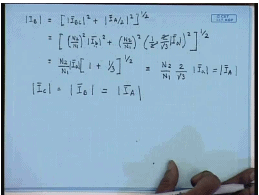Therefore, the phase current I B magnitude will equal to mod I b C square plus mod I A by 2 square to the power half, but I b C we have seen it to be N 2 by N1 square mod I b square and I A by 2 plus I A by 2 is N 2 by N 1 square into half into 2 by root 3 mod I a square to the power half. This is equal to N 2 by N 1 into; now in a balanced two phase system mod I B is same as mod I A. So, I can say this is equal to I a, 1 plus one-third to the power half. This is equal to N 2 by N 1 2 by root 3 mod of I a. This is same as the mod of I capital A. Similarly, it can be shown that mod of I C equal to mod of I B equal to mod of I A.

The angle between them is also 120 degree. This can be seen very easily. This is the phasor I A; this is the phasor I B, and this is the phasor I A by 2, but mod of I B we have just shown to be equal to I A. So, this length is half of this length. Hence, this angle is 30 degree, but this angle is 90 degree. Hence, this angle is 120 degrees. Similarly, this angle is also 120 degrees. So, we see when we put a balanced two phase load on the Scott connected transformer secondary of arbitrary power factor, it does not necessarily have to be unity power factor, then the three phase side currents are also balanced. What happens when we connect an unbalanced load?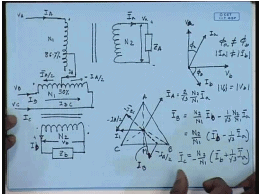Let us draw the same circuit diagram. Now we have connected an unbalanced load Z A to the voltage V a, the current is again I A and another load Z B the source V b. The currents drawn here is I capital A, I B, I C. We can draw the phasor diagram in a similar manner. On the secondary side, the voltages are still balanced though, because the supplied three phase voltage is balanced. So, this is V a, this is V b. Now let us say this is I a, and this is I b. They are unbalanced both in magnitude and in phase angle. This is phi a, this is phi b, unbalanced; hence, phi a is not equal to phi b and mod I a is not equal to mod I b, but nevertheless mod V a is still equal to mod V b.

So, let us see on the three phase side, what will happen? This is the three phase voltage phasor line voltage phasors A, B, C. This is M, this is, say, N. So, as in the previous case the line current will be I A, if this will be still be 2 by root 3 N 2 by N 1 into I small a. This will still be I b C. This is minus I b C and I B is I b C minus I A by 2. This will be the current I B and I C will be minus I b C minus I A by 2; obviously, the three phase currents are no longer balanced, but still they will be given by the same formula.

I B will be I b C which is N 2 by N 1 I B minus 1 by half I a minus I a by 2. This is I A; this is minus I A by 2; this is I A by 2. This current is I b C; this current is I b. I a by 2, hence it is 1 by root 3 N 2 by N 1 I a; that is equal to N 2 by N 1 I b minus 1 by root 3 I a. Similarly, I C will be minus of N 2 by N 1 I b plus 1 by root 3 I a. How much this magnitudes will be, let us try to see with an example.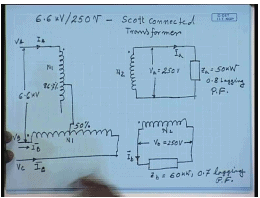Say, we have a 6.6 kV by 250 volts Scott connected transformer the leading phase that is this is the 6.6 kV. This is N 1; this is N 1 at 50 percent tapping, at 86.7 percent tapping. The line voltage and this is V A, V B, V C. The line voltage here is 6.6 kV, and this is the two phase side. This is V small a nominally this is 250 volts. Similarly, this number of turns is N 2 here. Here this is also N 2; this is also V B equal to 250 volts nominally.

Now the load on this transformer on the leading phase that is on phase A, I have connected a load Z a which is equal to nominally 50 kilowatt; that is when the voltage is 250 volts, it is 50 kilowatt at 0.8 lagging power factor. And I have connected another load Z b to the lagging phase. This current is I a; this current is I b equal to this is 60 kilowatt at 0.7 lagging power factor; that is the load is unbalanced. So, neglecting no load current we are expected to find out what will be the three phase input currents I A, I B and I C.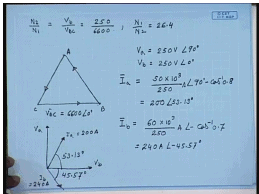Obviously, the turns ratio N 2 by N1 equal to V b by V b C equal to 250 divided by 6600 or N 1 by N 2 equal to 26.4. Now if we choose V b C to be the reference phasor, this is 6600 angel 0 degree. This is V b C; this is V a B; this is V c A. V b C is the reference phasor, then we know V a equal to 250 volts angle 90 degree and V B equal to 250 volts angle 0 degree. Therefore, the load currents I a equal to 50 kilowatt divided by this is 50 KVA and by mistake this is 50 KVA at 0.8, and this is 60 KVA at 0.7.

So, the load current I a is 50 KVA divided by 250 at an angle of 90 minus cos inverse 0.8 or I a equal to 200 amperes at an angle of 53.13 degrees. Similarly, phasor I b equal to 60 KVA divided by 250 amperes at an angle of minus cos inverse 0.7; that is I b equal to 240 amperes at an angle of minus 45.57 degrees. So, this is V a; this is V b. This is I a equal to 200 amperes, and this angle is 53.13 degrees. This is I b equal to 240 amperes, and this angle is 45.57 degrees. So, what will be the line current capital I A?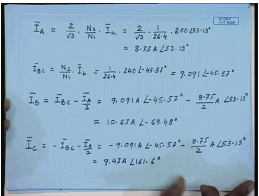Capital I A we have seen is given by 2 by root 3 into N 2 by N 1 into I a. Hence, this comes to 2 by root 3 into 1 by 26.4 into 200 angle 53.13 degrees. This comes to 8.75 ampere at an angle of 53.13 degrees. Now I b C will be equal to N 2 by N 1 into I B. Hence, this will be 1 by 26.4 into 240 angle minus 45.57 degrees. This comes to 9.091 angle minus 45.57 degrees.

Therefore, the current I B will be equal to I b C minus I A by 2; that is 9.091 ampere angle minus 45.57 degrees minus 8.75 by 2 amperes angle 53.13 degrees. Once we do the calculation it comes to 10.67 ampere angle of minus 69.48 degrees. The phasor I C will be minus I b C minus I a by 2; that is minus 9.091 ampere angle minus 45.57 degrees minus 8.75 by 2 amperes angle. This comes to 9.47 amperes angle 161.6 degrees.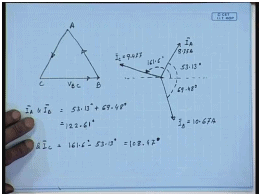So, if we draw the phasor diagram we have taken V b C to be the reference phasor, the current I a is 8.75 amperes at an angle of 53.13 degrees. This is the reference direction. So, this is let us say current I A. This angle is 53.13 degrees. The current I B is 10.67 ampere at an angle of almost minus 70 degrees. So, this is I B. This angle is 69.48 degrees; the magnitude is 10.67 amperes. On the other hand, I C is somewhere here; magnitude is 9.47 amperes, and this angle with respect to reference is 161.6 degrees. So, what is the angle between different? For example, between I A and I B the angle is 53.13 degrees plus 69.48 degrees. This comes to between I A and I B this angle is 122.61 degrees.

Similarly, what is the angle between I C and I A? This angle is 161.6 degrees minus 53.13 degrees; this angle is 108.47 degrees. So, we see that when the load current is unbalanced, the current supplied by the three phase side in a Scott connected transformer is also unbalanced. Now one extreme case of unbalance is of course, a single phase load; a Scott connected transformer can be used to supply a single phase load.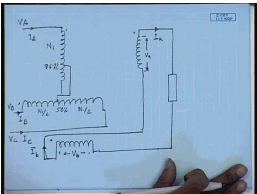Same connection, this is 50 percent tapping; this is 86.7 percent tapping. For single supplying single phase load, we connect these two points. We connect the windings in series, and this is how the single phase load is connected. So, the current here is I A; this is the current I b. If we look at the phasors this is the voltage V b; this is the voltage V a.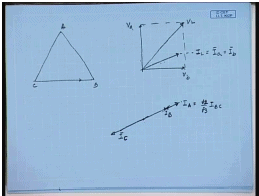If we look at the phasor diagram this is the input side phasor; this is V b; this is V a. The load voltage is V L, I a equal to I B equal to I l here. This is V b; this is V a. Hence, the load voltage is the vector sum of V a plus V b which is here this is the load voltage V L, and let us say this is the load current I L equal to I A equal to I B. Hence, we see that this is a special case of unbalanced loading where the magnitudes of the two phase currents are same, but their phase angles are not same.

So, what will happen here? The current I capital A will still be in the direction of I L, but now I b C which is, this will also be the direction of I b C. This will actually be root 3 by 2 times I b C, 2 by root 3 times. So, I B will be I b C minus I A by 2. So, I B will be somewhere here, and I C will be minus I b C minus I A by 2; I C will be somewhere here. So, even in the three phase side the line currents will be all in phase; that is what happens when a Scott connected transformer secondary’s are connected in series to supply a single phase load.

Offer running on EduRev: Apply code STAYHOME200 to get INR 200 off on our premium plan EduRev Infinity!

61 docs|20 tests

,

,

,

,

,

,

,

,

,

,

,

,

,

,

,

,

,

,

,

,

,

;